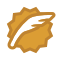# 【剑指Offer】56.2 数组中只出现一次的数字，其他出现3次

## 思路

### int位存储

``class Solution {public int singleNumber(int[] nums) {// 存储int 4*8=32每一位int[] arr = new int;for(int x : nums) for(int i = 0; i < 32; i++) {arr[i] += x & 1;x >>= 1;// 从低到高}int res = 0, mod = 3;for(int i = 0; i < 32; i++) {res <<= 1;// 从高到低res += arr[31-i] % mod;}return res;}}``

### 编译 自动机状态转移

``class Solution {public int singleNumber(int[] nums) {int one = 0, two = 0;// {one, two}每三个状态一次转移// {0, 0} {1, 0} {0, 1} {0, 0}for(int x : nums){one = (one ^ x) & ~two;two = (two ^ x) & ~one;}return one;}}``瑞 新CSDN认证博客专家分布式Java架构求职中 • Java全栈养成计划